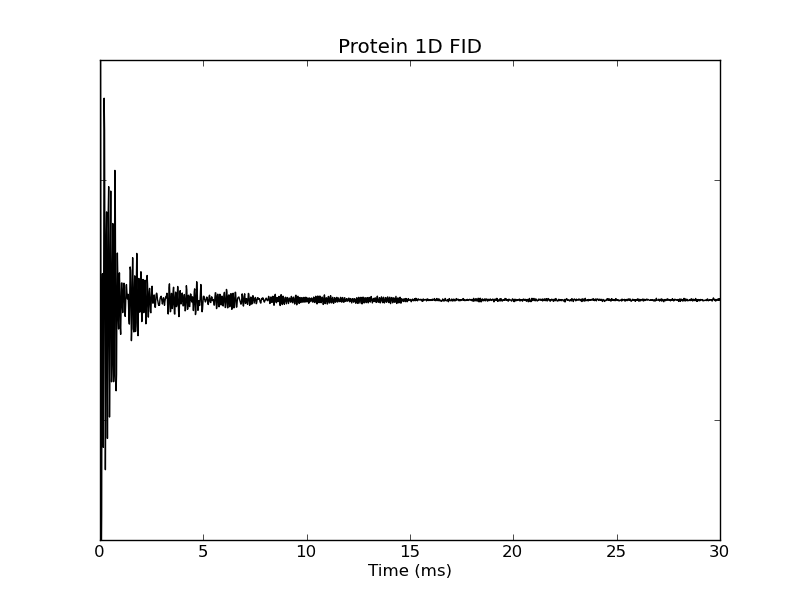# plotting example: plot_1d_time

This example shows how to use nmrglue and matplotlib to create figures for examining data or publication. In this example the fid from a 1D NMRPipe file is plotted.

```#! /usr/bin/env python
# Create a 1D plot of NMRPipe data

import nmrglue as ng
import matplotlib.pyplot as plt
import numpy as np

# read in the data from a NMRPipe file

# make a unit conversion object
uc = ng.pipe.make_uc(dic, data)

# plot the spectrum
fig = plt.figure()
ax.plot(uc.ms_scale(), data, 'k-')

# decorate axes
ax.set_yticklabels([])
ax.set_title("Protein 1D FID")
ax.set_xlabel("Time (ms)")
ax.set_ylim(-100000, 100000)

# save the figure
fig.savefig("fid.png") # this can be to .pdf, .ps, etc
```

Results: